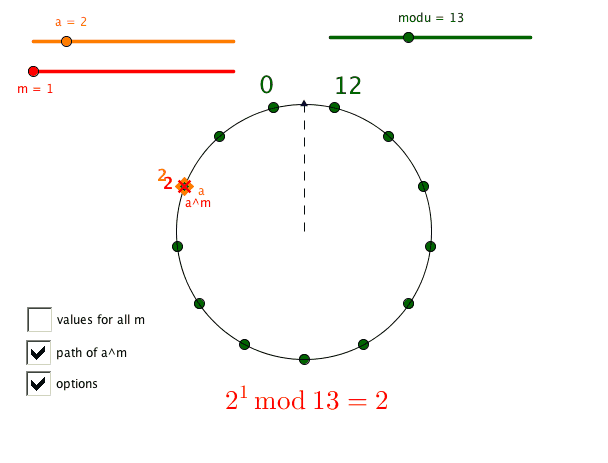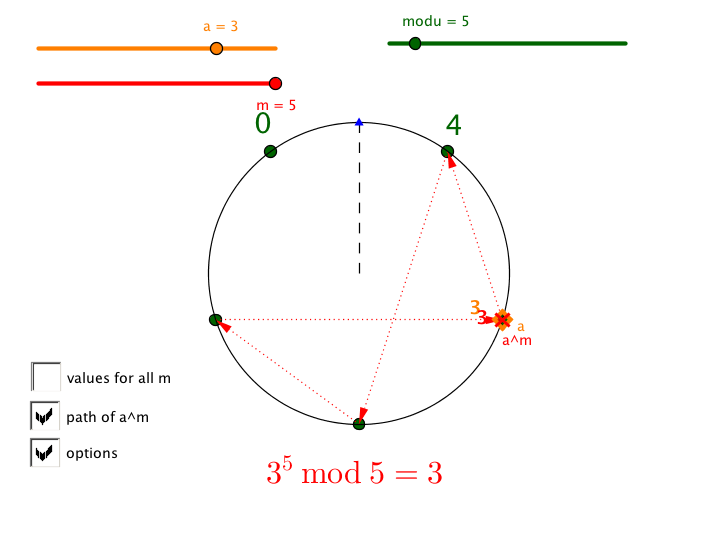## Friday, May 16, 2014

### I See Number Theory

We had a really interesting week in my number theory class. We are really a seminar, seven teachers and I investigating elementary number theory together.  I hope they're learning half as much as I am.

This week we were exploring primes and modular arithmetic. The first day we were thinking about the $$4x \pm 1$$ and $$6x \pm 1$$ structures, and the results that there are an infinite number of each type of prime.

To gain modular arithmetic practice, we played Modular Skirmish. (Cf. this post on Gauss.)

Then we started looking at this GeoGebra sketch:

The numbers increase from the bottom left corner up the column. My first attempt was a growing square, but that let you see asymptotic distribution of primes more than the modular structure.

We put this sketch up on the front screen, and advanced n. Teachers noticed the empty top rows (multiples of the modulus) and how some values separated the primes into rows: 2, 4, 6, 8, 10, 12... while others seemed to form diagonals: 3, 5, 7, 11, 13... We wondered about which were the most consecutive primes or gaps in a row, and whether that would change as m increased. (Personally I got wondering about where are the largest square gaps.) Teachers connected many of the patterns to the rows in 6. For example, in 7:
The diagonal really means the next prime is +7 -1 or 6 apart.So it goes back to that 6 structure.

Modulo 10 is really just looking at the last digits. We noticed that no digit seemed more or less common out of the four possible. Also, no consecutive dots more than 2. Is that always true?

The two coolest structure theorems are with respect to four and six. I think these helped in understanding why primes are of the form $$4x \pm 1$$ or $$6x \pm 1$$. Which may have also helped with the proof that there are an infinite number of primes of the form $$4x - 1$$ (or $$6x - 1$$ ).

We did find a modulus where there was a row of 8 consecutive primes, but I can't rediscover it!

Understanding the six structure also helped us understand a diagram that we were looking at the previous week, from a designer who was really impressed with a 12 structure. (Source in reddit/r/mathpics. The picture isn't super precise, but did offer a lot of making sense opportunities. And colorful!)

Rather than make the course a tour through the great theorems of number theory, my hope is that it can be an opportunity to do math ourselves. So instead of necessarily illustrating a theorem, I'd rather find a way to notice things that might lead to the theorem. Since we're interested in K-12 applications, divisibility tests and primality tests are of interest; that means exploring the ideas in Fermat's Little Theorem.

So the idea came - given the success of the modality/primes visualization - to visualize exponential patterns in the modular context. This sketch is what I came up with.Oh! The patterns they found!

Definitely a lot of things that I had not noticed. Not, interestingly, Fermat's Little Theorem, but there were many observations that will lead there.

A lot of our discussion was about pairs of cycles. The visualization made it clear when two different bases created the same path, up to direction. Eg. $$2^m \mod 5$$ and $$3^m \mod 5$$.Furthermore, they noticed this awesome pairing within the cycles. Here's the nicest mod 13 pair.

Look back at the other data... there's a lot to notice. And it definitely has me wondering. (Copyright, trademark and kudos to Max and Annie from the Math Forum.)

It's hard to imagine that introducing a theorem and sharing a proof would have resulted in building any more understanding, and there's no way it would have led to doing any more math. And this will make the theorem so much more meaningful when we get there. If we do, with such a fine boatload of conjectures to explore.

1.I have a Processing.js version of your GeoGebra circle: http://amathknauft.com/visuals/sketch.php?powers-modulo2

There are so many patterns to be seen! The pairing are especially easy to spot when you can see all M starting bases cycling through their powers at the same time. I've got a related sketch that was designed to visualize quadratic reciprocity that you also might be interested in: http://amathknauft.com/visuals/residues.html

2.Thanks for sharing those - they're super sweet! The residue sketch in particular is a wonder.

3.It's tricky to envision that presenting a hypothesis and imparting a verification would have brought about building any additionally understanding, and it is extremely unlikely it would have prompted doing any more math. What's more this will make the hypothesis such a great deal more significant when we get there.
Free Online 6th Grade Math Practice